+

# Mean, Median, and Mode

Author: Sophia Tutorial
##### Description:

Calculate the mean, median, or mode of a distribution.

(more)

Sophia’s self-paced online courses are a great way to save time and money as you earn credits eligible for transfer to many different colleges and universities.*

No credit card required

46 Sophia partners guarantee credit transfer.

299 Institutions have accepted or given pre-approval for credit transfer.

* The American Council on Education's College Credit Recommendation Service (ACE Credit®) has evaluated and recommended college credit for 33 of Sophia’s online courses. Many different colleges and universities consider ACE CREDIT recommendations in determining the applicability to their course and degree programs.

Tutorial
what's covered
This tutorial will cover how to calculate the mean, median, and mode of a data set. Our discussion breaks down as follows:

1. Mean
1. Calculating Mean
2. Outliers
3. Notation
2. Median
1. Calculating Median
2. Extreme Values
3. Median Class
3. Mode
1. Qualitative Sets
2. Distributions

## 1. Mean

The word mean is often used interchangeably with the word “average.” However, several different things can be called an average; the mean is the most common of those. In this tutorial, "mean” will be used interchangeably with the word “average,” whereas other concepts, such as median, will not be implied.

term to know

Mean
The "average" value of a data set. It is obtained by dividing the sum of the values by the number of values in the set.

1a. Calculating Mean

The mean of a data set is found by adding up all the values together and dividing by how many there are. Notationally, it looks like this:

formula
Mean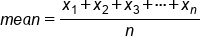The 1's, 2's, and 3's in the X₁ X₂ X₃--also known as subscripts--indicate the first number in the list, the second number in the list, and so forth, until the last number in the list, marked by the Xn. The “n” value in the denominator is the total number of values.

EXAMPLE

The data set below shows the height of the players on the Chicago Bulls basketball team. What height would be considered average height for basketball players on this team?

Player Height
Omer Asik 84
Carlos Boozer 81
Ronnie Brewer 79
Jimmy Butler 79
Luol Deng 81
Taj Gibson 81
Richard Hamilton 79
Mike James 74
Kyle Korver 79
John Lucas III 71
Joakim Noah 83
Derrick Rose 75
Brian Scalabrine 81
Marquis Teague 74
C.J. Watson 74

To find the answer, add all the values and divide by the total number of values that are represented. In this case, we're going to add up all of the players' heights and divide by 15, because there are 15 total players.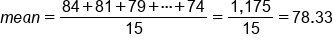The result is 78.33 inches, which is the average height of a player on the Chicago Bulls.
To calculate mean using technology, you can use a spreadsheet. Create your list of values and type “= average(”. Highlight all of the fields, close the parentheses, and hit “Enter”.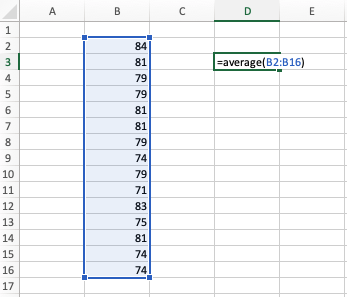In this example, the spreadsheet will return with 78.33, which is the same number you calculated in your notation above.

1b. Outliers
You may come across situations in which the mean is a poor representation of where the center actually is.

EXAMPLE

Suppose that you have 12 employees. Eight of them are shift workers, three of them are managers, and there’s one boss. Here are the salaries for the respective positions:

Shift Worker: \$42,000
Manager: \$55,000
Boss: \$200,000

Calculate the mean of the eight shift workers, the three managers, and the boss.This means that the mean of the 12 workers is over \$58,000. However, how many of the employees actually make more than \$58,000? How many make less than \$58,000?
11 of the 12 employees make less than \$58,000, and only one makes more than that and that one person makes substantially above that amount. Therefore, it doesn't really make a lot of sense to measure center. The boss’s \$200,000 salary is an outlier in this data set.

hint
In the presence of outliers, which are very few high or very few very low values, the mean won't give an accurate representation of center.

1c. Notation
There are a couple of accepted notations for expressing averages:

Symbol Pronunciation Description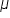"mew" Is a Greek letter; you will see this quite a lot as a notation for the mean.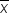“x-bar”: This is simply an x with a bar over it; you can also use a y-bar, or whatever value you're using.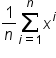"sigma" Is a Greek letter; called summation notation

Summation notation, or sigma notation, is a different special notation to shorten up all of this summation. This notation uses the Greek letter, sigma: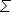. The compact formula of the summation notation is the same as the lengthier formula.

formula
Mean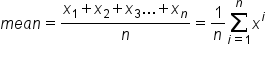The Xᵢ (read as “x subscript i”) is just like the X₁, X₂, and X₃ in the original summation (from the first section). Therefore, this notation means that the value of Xᵢ will be the sum of all the X's, starting from the first one (where the i value is 1) and finishing at the “nth,” or last, one. When that is completed, you divide by n.

term to know

Summation Notation
A notation that uses the Greek letter sigma to state that values should be added together.

## 2. Median

A median is simply a measure of center for the data set that actually finds the middle value in a sorted list. It's the middle number when the data set is arranged from least to greatest or greatest to least.

term to know

Median
The value that is in the "middle" of a data set when the set is arranged from least to greatest.

2a. Calculating Median

Recall the list heights of players from the Chicago Bulls basketball team.

Player Height
Omer Asik 84
Carlos Boozer 81
Ronnie Brewer 79
Jimmy Butler 79
Luol Deng 81
Taj Gibson 81
Richard Hamilton 79
Mike James 74
Kyle Korver 79
John Lucas III 71
Joakim Noah 83
Derrick Rose 75
Brian Scalabrine 81
Marquis Teague 74
C.J. Watson 74

You might notice that many of the players are 81 inches tall. Can you, therefore, call that height typical of the Chicago Bulls? To answer that question, you'll need to calculate the median.

In the above list, the players were sorted alphabetically. To find the median, you need to have that list ordered from least to greatest. The first step, therefore, is to reorder those numbers, which will look like this:

71, 74, 74, 74, 75, 79, 79, 79, 79, 81, 81, 81, 81, 83, 84

To find the middle number, start by crossing off the lowest and highest numbers and continue working your way in until you have just one number left.

71, 74, 74, 74, 75, 79, 79, 79, 79, 81, 81, 81, 81, 83, 84,

In this case, the remaining number is 79, which is the median. Notice that half the values in the list are at or below 79, and half the values in the list are at or above 79.

You can also use technology to figure out the median of a data set. Place the list of heights, not ordered, in a spreadsheet. Type "= median(". Then, select the full range of numbers for which you want to find the median, close the parentheses, and hit "Enter".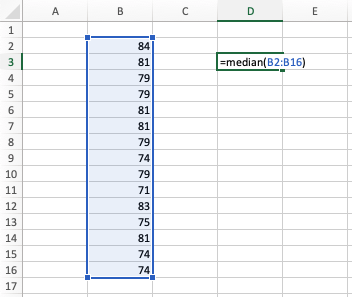Using this method, your spreadsheet will give you a median of 79, just like the first method above.

If you have an even set of data, such as 16 pets or 20 courses, finding the median will take an extra step.

EXAMPLE

Suppose you have a class of 10 students and you have a 10-point quiz. Below are the scores from each of the students. What is the median?

10, 9, 6, 7, 7, 8, 9, 9, 10, 4, 7, 8

The first step is to reorder these scores, which should result in a list that looks like this:

4, 6, 7, 7, 7, 8, 9, 9, 9, 9, 10, 10

As you cross out the highest and lowest numbers, working toward the center, you will notice that there are two middle values:

4, 6, 7, 7, 7, 8, 9, 9, 9, 9, 10, 10,

In a case like this, you have to average those two numbers by adding the values together and dividing by 2: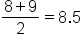Therefore, the median is 8.5.

2b. Extreme Values
How is the median affected by extreme values? Suppose that you have another 10-point quiz for a different class of 11 students. Here are the scores, in order:
2, 4, 5, 6, 6, 7, 8, 8, 8, 9, 90

The median for this set of data is 7.

Obviously, one of these values, 90, is completely out of range, perhaps because of a typo. Despite this typo, however, the median of this data set is 7 because that is the middle number. If you correct the typo, changing that 90 to a 9, for instance, the median will still be 7.

big idea
The median is not overly affected by outliers or extreme values.

2c. Median Class
Another way to figure out a median is to use data summarized in a frequency table, which can help you find the median class.

When is it best to use a frequency table? Let's explore an example. Here is information about the number of days that the temperature was in a particular range in Chanhassen, Minnesota in 2009:

Temperature Frequency Cumulative Frequency Relative Cumulative Frequency
-10 - -1 3 3 0.01
0 - 9 8 11 0.03
10 - 19 25 36 0.10
20 - 29 39 75 0.21
30 - 39 30 105 0.29
40 - 49 51 156 0.43
50 - 59 46 202 0.55
60 - 69 39 241 0.66
70 - 79 80 321 0.88
80 - 89 40 361 0.99
90 - 99 4 365 1.00

You can see, for example, that eight days had a temperature of between 0 and 9 degrees Fahrenheit. Using this table, there are a couple of different ways to find not exactly what the median temperature is, but which bin it's in.

hint
There are 365 days in a year, so the median would be on Day 183. There are 182 days before this date, and 182 days after this date, for a total of 365 days

Temperature Frequency Cumulative Frequency Relative Cumulative Frequency
-10 - -1 3 3 0.01
0 - 9 8 11 0.03
10 - 19 25 36 0.10
20 - 29 39 75 0.21
30 - 39 30 105 0.29
40 - 49 51 156 0.43
50 - 59 46 202 0.55
60 - 69 39 241 0.66
70 - 79 80 321 0.88
80 - 89 40 361 0.99
90 - 99 4 365 1.00

You can see that the 183rd day of the year falls in the 50-59 category. That means that 182 days were as cold or colder than that particular day, and 182 days were at least as warm as that particular day, which means that these are semi-ordered by temperature. Thus, the median is somewhere in the 50's.

You can't be 100% sure exactly where in the 50's it is, but you can be sure that it's in the 50's. Notice the number 183, when you look at the cumulative frequency, falls between the 156 and the 202. By the time you've gotten to the end of the temperatures in the 40's, you haven’t accounted for half the days in terms of ordered temperatures. But by the time you finish the 50's, you have accounted for more than half the days, which means that the median is somewhere in the 50's.

If you look at the relative cumulative frequency column, you can see the same thing. By the time you have finished the 40's, you've accounted for less than 43% of the data. By the time you finish the 50's, however, you will have accounted for over 55% of the data.

Where's the 50th percentile? You don't know what the number is, but again, you know it's somewhere in the 50's: 50% of days fall in or below the 50's. Therefore, you would call the 50's the median class because you know the median is somewhere in that bin.

term to know

Median Class
The bin that contains the median value. This is the most precise measurement we can obtain when we are looking at data that have already been categorized.

## 3. Mode

There are a couple of different ways to go about determining what would be considered typical for a set of data:

• Mean, which would require adding all of these numbers up and dividing by 15.
• Median, which would require ordering the values from least to greatest and find the number that's in the middle.
• Mode, which is the value that appears most frequently

In a quantitative set, the mode is the most frequently occurring number or numbers, assuming that they occur more than once.

EXAMPLE

Recall our list from above detailing the heights of the Chicago Bulls basketball team. Using this list, can you find out what height would be considered the mode?

Player Height
Omer Asik 84
Carlos Boozer 81
Ronnie Brewer 79
Jimmy Butler 79
Luol Deng 81
Taj Gibson 81
Richard Hamilton 79
Mike James 74
Kyle Korver 79
John Lucas III 71
Joakim Noah 83
Derrick Rose 75
Brian Scalabrine 81
Marquis Teague 74
C.J. Watson 74

In our data set of heights, you can see that 81 occurs four times, and 79 also occurs four times. Unlike with means and medians, a distribution can have more than one mode. In this case, 79 and 81 are both modes.

try it
Suppose a class has 12 students. Here are the grades from a 10-point quiz. Determine the mode.

10, 9, 6, 7, 7, 9, 9, 9, 10, 4, 7, 8

You probably realize that the mode is nine--it appears four times, and nothing else appears more than three times.
term to know

Mode
The most frequently appearing number in a set of quantitative data or most frequently occurring category in a set of qualitative data.

3a. Qualitative Sets

You can also find the mode of a qualitative data set. In a qualitative data set, the mode is the most frequently occurring category or the largest category.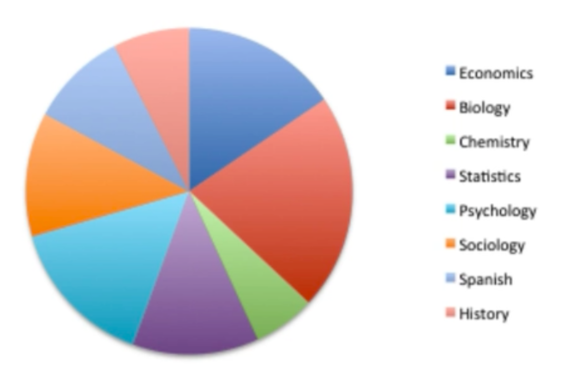In the pie chart above, there are several large categories, but the largest category is the red one: biology. Therefore, biology would be the mode of this data set.

3b. Distributions
In a distribution that is fully graphed out, you might have something that's multi-peaked, like in the graph below.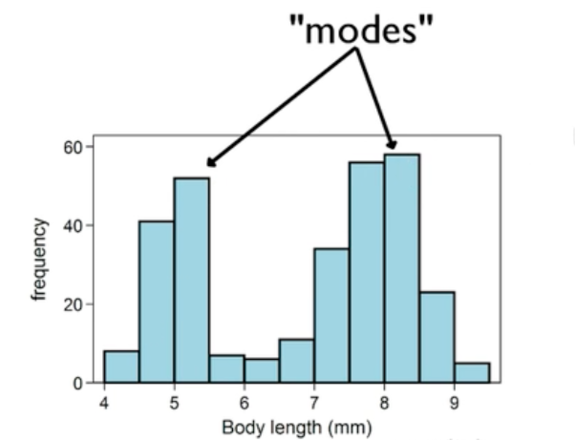As this graph shows, there is a gap between the two highest peaks, where the amounts decrease very precipitously and then rise again. In a distribution, we would call both of these areas modes. The values near five and eight would both be considered modes because they are the different peaks in the distribution. It might still be called bimodal, although in reality there is only the one mode, the highest bar at eight.

summary
The mean is one measure of center that we can use, and it's what is meant by the term “average.” When measuring mean, it is important to consider outliers, which are very few high or very few very low values. If you factor in outliers, the mean won't give an accurate representation of center. Sometimes, summation notation can be used as a shortcut instead of writing the whole long string of added values.

The median identifies the middle number in a set of ordered data. If there's an even number of data values, you take the mean of those two middle numbers. Even for data sets with extreme values, the median will still be the middle number. If the data is on a frequency table, you can find the median class, but you can't find the exact median itself.

The mode is the most common value in a data set. In qualitative data sets, that value can be a category, and in a quantitative data set, the value will be a number. There can be one mode, many modes (if several values appear an equal amount of plural times), or no mode if no value appears more than one time. Modes may also refer to the peak or peaks of distributions, even if they're not the tallest point in the distribution. If a distribution has many peaks, they can be called bimodal or multimodal.

Good luck!

Source: Adapted from Sophia tutorial by Jonathan Osters.

Terms to Know
Mean

The "average" value of a data set. It is obtained by dividing the sum of the values by the number of values in the set.

Median

The value that is in the "middle" of a data set when the set is arranged from least to greatest.

Median Class

The bin that contains the median value. This is the most precise measurement we can obtain when we are looking at data that have already been categorized.

Mode

The most frequently appearing number in a set of quantitative data or most frequently occurring category in a set of qualitative data.

Summation Notation

A notation that uses the Greek letter sigma to state that values should be added together.

Weighted Mean/Average

A way of calculating a mean when not all the values count for the same amount. Each value should be multiplied by its weight and added together, then divide the sum by the sum of the weights.

Formulas to Know
MeanRating